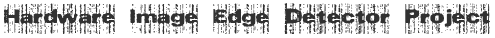Edge Detection in FPGA using Sobel Operator

### Edge Detection Theory

An edge can be defined as an abrupt change in brightness as we move from one pixel to its neighbour in an image. In digital image processing, each image is quantized into pixels. With gray-scale images, each pixel indicates the level of brightness of the image in a particular spot: 0 represents black, and with 8-bit pixels, 255 represent white. An edge is an abrupt change in the brightness (gray scale level) of the pixels. Detecting edges is an important task \in boundary detection, motion detection/estimation, texture analysis, segmentation, and object identification.
Edge information for a particular pixel is obtained by exploring the brightness of pixels in the neighborhood of that pixel. If all of the pixels in the neighborhood have almost the same brightness, then there is probably no edge at that point. However, if some of the neighbors are much brighter than the others, then there is a probably an edge at that point. Measuring the relative brightness of pixels in a neighborhood is mathematically analogous to calculating the derivative of brightness. The image illustrates an example of Hard and Soft Edges on an image. Brightness values are discrete, not continuous, so we approximate the derivative function. Different edge detection methods (Prewitt, Laplacian, Roberts, Sobel and Canny) use different discrete approximations of the derivative function.
For example consider a random discrete 9 x 9 pixel image.
Refresh the page to see randomize the pattern. Horizontal Edge Example Vertical Edge Example Diagonal Edge Example
X difference is calculated as | I(i+1,j) - I(i,j)| Y difference is calculated as | I(i,j+1) - I(i,j)| where I denotes the intensity values [0-255]

more »

### Sobel Edge Detection Operator

The Sobel Edge Detection Operator is a 3x3 Spatial mask. It is based on the differential operation [1 0 -1] and an averaging operator [1 2 1], Convolving these operators we get the 3x3 spatial mask for sobel:
-1 0 1 Horizontal Gradient Operator -2 0 2 -1 0 1
-1 -2 -1 Vertical Gradient Operator 0 0 0 1 2 1
The Spatial mask is convolved over the image to obtain the edge or high passed image. The image shows the convolution mask working over an image. The mask is convolved and the center element is replaced as the mask operates on the image. The borders of the images are usually blacked out, since they cannot be computed with a 3x3 mask, or partially computed.
Gx[i, j] = Im[i+1, j-1] + 2*Im[i+1, j] + Im(i+1, j+1) – { Im[i-1, j-1] + 2*Im[i-1, j] + Im(i-1, j+1) }
Gy[i, j] = Im[i-1, j+1] + 2*Im[i, j+1] + Im(i+1, j+1) – { Im[i-1, j-1] + 2*Im[i, j-1] + Im(i+1, j-1) }

more »